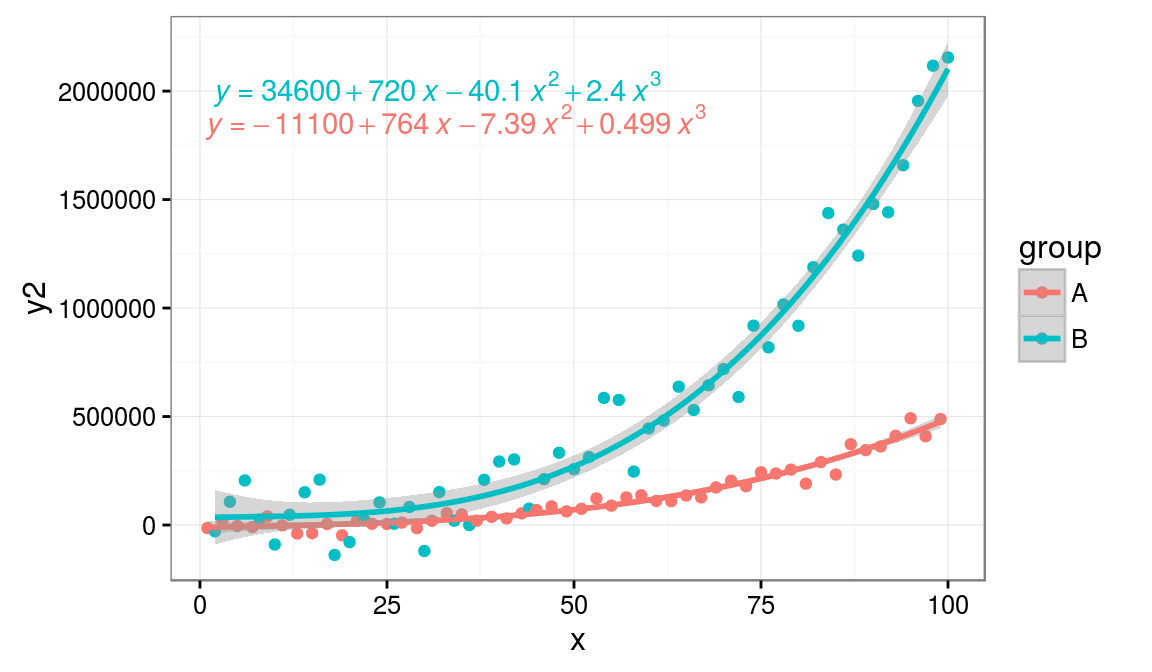ggplot2 now has an official extension mechanism. This means that others can now easily create their own stats, geoms and positions, and provide them in other packages. This should allow the ggplot2 community to flourish, even as less development work happens in ggplot2 itself. This page showcases these extensions.

### ggpmisc

https://cran.rstudio.com/web/packages/ggpmisc/

Miscellaneous Extensions to ‘ggplot2’.

``````# Example from https://cran.rstudio.com/web/packages/ggpmisc/vignettes/examples.html
library(ggplot2)
library(ggpmisc)

## stat_poly_eq()

## We generate some artificial data.
set.seed(4321)
# generate artificial data
x <- 1:100
y <- (x + x^2 + x^3) + rnorm(length(x), mean = 0, sd = mean(x^3) / 4)
my.data <- data.frame(x, y, group = c("A", "B"), y2 = y * c(0.5,2))

formula <- y ~ poly(x, 3, raw = TRUE)
ggplot(my.data, aes(x, y2, colour = group)) +
geom_point() +
geom_smooth(method = "lm", formula = formula) +
stat_poly_eq(aes(label = ..eq.label..), vjust = c(-8, 0),
formula = formula, parse = TRUE) +
theme_bw()``````